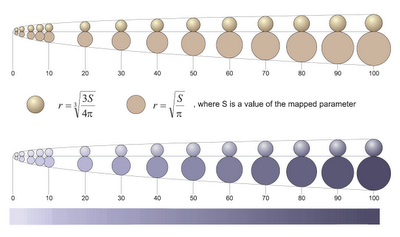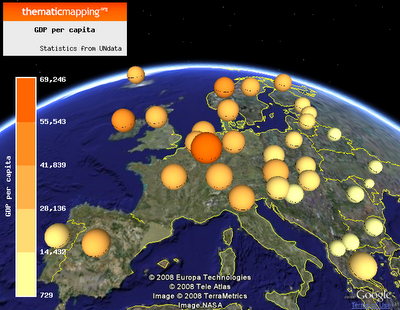Monday, 23 June 2008

Proportional symbols in three dimensions

Since I'm making my own Thematic Mapping Engine, I need to understand the math behind proportional symbol calculations. Originally, I thought I would need different equations for different geometric shapes, and my book in cartography gave me the same impression. But after reading this article, I realised that life was not that complicated.

This tutorial is a summary of a discussion on the CartoTalk forum. I especially want to thank Dominik Mikiewicz (mika) for his valuable comments and figures. The CartoTalk forum is highly recommended!

Equations for 1D, 2D and 3D proportional symbols:

1-dimensional symbols (height)
This is how the height of bars or prisms is calculated in TME.

Equation: symbolSize = (value / maxValue) * maxSize
PHP: \$symbolSize = (\$value / \$maxValue) * \$maxSize
JavaScript: symbolSize = (value / maxValue) * maxSize

Bars or prisms show “real” values scaled down to fit on a map, and you can easily see the relations and which is higher than the other. I’m not considering the problems caused by perspective and the curvature of the earth.

2-dimensional symbols (area)
This is how proportional images and regular polygons (e.g. circle, square) are scaled in TME.

Equation: symbolSize = power(value/maxValue; 1/2) * maxSize
PHP: \$symbolSize = pow(\$value/\$maxValue, 1/2) * \$maxSize
JavaScript: symbolSize = Math.pow(value/maxValue, 1/2) * maxSize

2D symbols use areas as mean of expression and therefore you're dealing with a square root of a showed value. This makes it relatively difficult to assess a value.

3-dimensional symbols (volume)
This is how 3D Collada objects (e.g. cube, sphere) are scaled in TME.

Equation: symbolSize = power(value/maxValue; 1/3) * maxSize
PHP: \$symbolSize = pow(\$value/\$maxValue, 1/3) * \$maxSize
JavaScript: symbolSize = Math.pow(value/maxValue, 1/3) * maxSize

3D objects use volumes as mean of expression so you’re showing a cube root of the value. This makes it difficult to assess a value.

It’s important to know that it’s one degree harder for the viewer to assess the relative size of 3-dimensional symbols compared to 3-dimensional, which again is harder to compare to 1-dimensional. This is clearly visualised on this figure (credit: Dominik Mikiewicz):The figure compares the circle and sphere radius for the same values.

These three images shows GDP per capita (2006, UNdata) using bars (1D), circles (2D) and spheres (3D).A colour scale and legend helps the user in assessing and comparing symbols.

The visual appearance of 2D and 3D symbols can also be improved by using a perceptual or logarithmic scale.